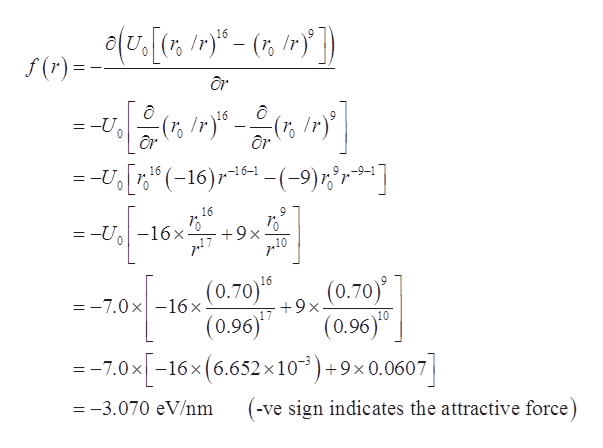# One model for the potential energy of a two-atom molecule, where the atoms are separated by a distance r, is U(r) = U0 [(r0 / r)16 - (r0 / r)9] where r0 = 0.70 nm and U0 = 7.0 eV. Note: 1 eV = 1.6*10-19 J. You can use SI units, but CAPA will also accept these units that will simplify your calculations: [Force] = eV/nm[Energy] = eV[distance] = nmr_eq = 7.6 *10^-1 nmIf the distance between the atoms increases from equilibrium by r1 = 0.20 nm, then what is the force from one atom on the other associated with this potential energy? (Enter your answer as postive if they repel each other, and negative if they attract). F_r (r_eq +r_1) = ?The atoms are oscillating back and forth. The maximum separation of the atoms is r2 = 2.2 nm. What is the kinetic energy of the atoms when they are separated by the equilibrium distance? K(r_eq) = ?

Question
13 views

One model for the potential energy of a two-atom molecule, where the atoms are separated by a distance r, is

U(r) = U0 [(r0 / r)16 - (r0 / r)9]

where r0 = 0.70 nm and U0 = 7.0 eV.
Note: 1 eV = 1.6*10-19 J.
You can use SI units, but CAPA will also accept these units that will simplify your calculations:
[Force] = eV/nm
[Energy] = eV
[distance] = nm

r_eq = 7.6 *10^-1 nm

1. If the distance between the atoms increases from equilibrium by r1 = 0.20 nm, then what is the force from one atom on the other associated with this potential energy? (Enter your answer as postive if they repel each other, and negative if they attract). F_r (r_eq +r_1) = ?
2. The atoms are oscillating back and forth. The maximum separation of the atoms is r2 = 2.2 nm. What is the kinetic energy of the atoms when they are separated by the equilibrium distance? K(r_eq) = ?
check_circle

star
star
star
star
star
1 Rating
Step 1

Given data:

ro = 0.70 nm = 0.70 x 10-9 m

Increased distance, r1 = 0.20 nm

req = 7.6 x 10-1 nm =  0.76 nm

r = req + r1 = 0.76 + 0.20 = 0.96 nm

U0 = 7.0 eV

1 eV = 1.6 x 10-19 J

Step 2

Formula used:

Step 3

Therefore...help_outlineImage Transcriptionclose16 /r f(r) =-U 16 9 ( /r) 0 --U (-16)4(-9)r ] -9-1 0 16 9 = -U-16x +9x (0.70) (0.96)T --7.0x-16x(6.652x10)+9x0.0607] 16 (0.70) (0.96)0 -7.0x16x +9x =-3.070 eV/nm (-ve sign indicates the attractive force) fullscreen

### Want to see the full answer?

See Solution

#### Want to see this answer and more?

Solutions are written by subject experts who are available 24/7. Questions are typically answered within 1 hour.*

See Solution
*Response times may vary by subject and question.
Tagged in

### Physics